# Introduction to missingHE

The package missingHE is specifically designed to facilitate the implementation of different types of missing data models to conduct trial-based health economic evaluations from a Bayesian statistical perspective. The main justification for the use of Bayesian models in economic evaluations is related to the decision-making nature of the problem, which imposes the need to assess the impact of different sources of uncertainty both on the statistical and cost-effectiveness results.

While frequentist methods are popular among practitioners, they require ad-hoc steps for quantifying the uncertainty around the estimated quantities of interest. Examples include the need to perform some form of bootstrapping to generate standard cost-effectiveness outputs (e.g. cost-effectiveness planes and acceptability curves), or the use of multiple imputation to account for missingness uncertainty. Although these steps can lead to statistically valid results, in practice, when the complexity of the model is increased (for example to deal with common statistical issues of the data such as correlation, clustering, missingness or skewenss), then the task of correctly combining all these steps may become incredibly difficult. The Bayesian approach, instead, allows to fit the model of interest while simultaneously handling all the different issues related to the data as well as to correctly propagate and quantify uncertainty for each unobserved quantitiy in the model (being a parameter or a missing value).

Different types of missing data models exist, each with its own advantages and disadvantages when it comes down to the strategy used to handle missingness. missingHE implements three types of models: selection models, pattern mixture models, and hurdle models. All models are implemented via Markov Chain Monte Carlo (MCMC) algorithms based on the BUGS language and the Bayesian program JAGS. For techincal details and an overview of the software see http://mcmc-jags.sourceforge.net/.

For each model, the package provides a series of ancillary functions for assessing convergence of the algorithm, checking the fit to the data, extracting and summarising the results. This brief tutorial aims at helping getting started with the package and its main functions. Throughout the document, we will use the built-in dataset called MenSS as a toy example, which is directly available when installing and loading missingHE in your R workspace. It is important to remember that missingHE only allows the analysis of two-arm studies (comparison of more than two intervantions is not currently supported) and is designed to handle missing values only in the outcome variables (no missing values in the covariates are allowed).

If you would like to have more information on the package, or would like to point out potential issues in the current version, feel free to contact the maintainer at [email protected]. Suggestions on how to improve the package are also very welcome.

## Data: MenSS

The Men’s Safer Sex (MenSS) trial was a pilot randomised controlled trial conducted on young men at risk of sexually transmitted infections (STIs). A total of $$159$$ individuals were enrolled in the trial, $$75$$ in the comparator group ($$t=1$$, standard of care) and $$84$$ in the reference group ($$t=2$$, standard of care plus online intervention). Clinical and health economic outcomes were collected via self-reported questionnaires at baseline, $$3$$, $$6$$, and $$12$$ months follow-ups.

• Health economic data included utility scores based on health-related quality of life (from EQ5D-3L) and costs based on resource use information (from CSRI). QALYs and Total costs were derived from aggregating the utility and cost data using the area under the curve method and by summing up the follow-up costs over the trial duration, respectively.

• Clinical data included the number of instances of unprotected sex and whether or not each individual was associated with an STI diagnosis.

The dataset MenSS includes the main variables of interest for the economic evaluation: the individual-level QALYs and baseline utilities, Total costs, as well as the number of instances of unprotected sex and the STI diagosis indicator at baseline and $$12$$ months follow-up. Additional baseline variables provided are: id, treatment indicator, age, ethnicity, employment status and site. We can display a summary description of the variables in MenSS by using the str command

> str(MenSS)
#> 'data.frame':    159 obs. of  13 variables:
#>  $id : int 1 2 3 4 5 6 7 8 9 10 ... #>$ u.0       : num  0.725 0.848 0.848 1 0.796 ...
#>  $e : num NA 0.924 NA NA NA 0.943 NA NA NA 0.631 ... #>$ c         : num  NA 0 NA NA NA 0 NA NA NA 516 ...
#>  $age : int 23 23 27 27 18 25 48 30 24 27 ... #>$ ethnicity : Factor w/ 2 levels "0","1": 1 1 2 1 1 2 2 2 2 1 ...
#>  $employment: Factor w/ 2 levels "0","1": 1 2 1 1 1 2 2 2 2 2 ... #>$ t         : int  1 1 1 1 1 1 1 1 1 1 ...
#>  $sex_inst.0: num 25 30 6 20 1 20 6 4 1 12 ... #>$ sex_inst  : int  NA 50 3 0 99 NA 20 NA NA NA ...
#>  $sti.0 : int 0 0 0 0 0 1 0 0 1 0 ... #>$ sti       : int  1 0 0 0 0 0 0 0 0 0 ...
#>  $site : Factor w/ 3 levels "1","2","3": 1 3 3 1 3 1 1 1 1 2 ... We can look at the empirical distributions of the data, for example the QALYs ($$e$$) and Total costs ($$c$$) variables, by treatment arm to have a general idea about what type of issues seem to be relevant for our analysis. Remember that, when using your own dataset, variables must be assigned specific names when using the functions from missingHE. In particular, the treatment arm indicator should take value $$1$$ and $$2$$ for the control and intervention group, while the effectiveness and cost variables should be assigned the names $$e$$ and $$c$$, respectively. > par(mfrow=c(2,2)) > hist(MenSS$e[MenSS$t==1], main = "QALYs - Control") > hist(MenSS$e[MenSS$t==2], main = "QALYs - Intervention") > hist(MenSS$c[MenSS$t==1], main = "Costs - Control") > hist(MenSS$c[MenSS$t==2], main = "Costs - Intervention")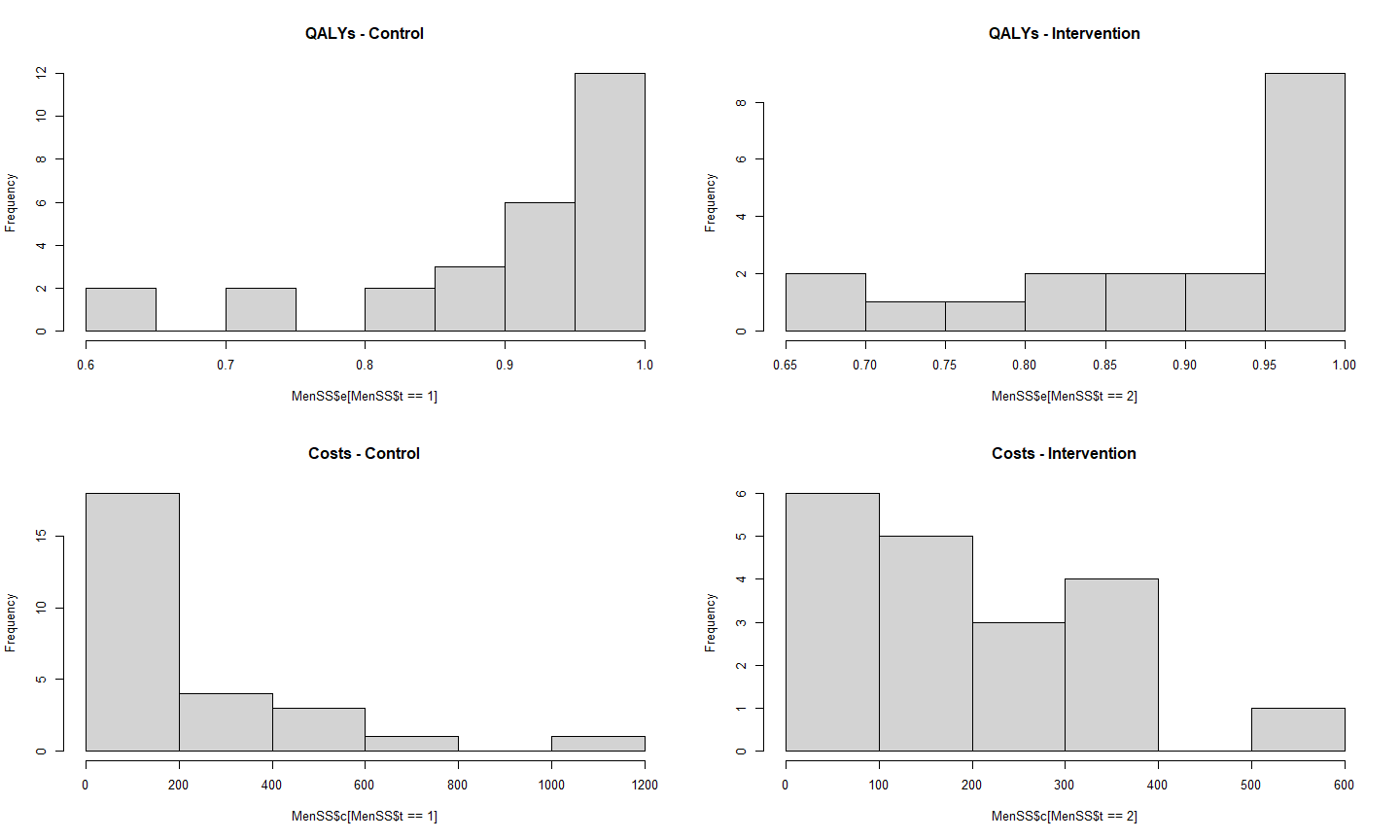We can immediately see that non-normality seems to be relatively high in both the QALYs (negatively skewed) and Total costs (positively skewed) in each treatment group. In addition, there is a considerable amount of identical or structural values for both outcomes (ones for QALYs and zeros for costs), which increases the degree of skewness in the data. The proportions of these structural values by type of outome and treatment group can be extracted using the following commands > #proportions of ones and zeros in the control group > c(sum(MenSS$e[MenSS$t==1]==1, na.rm = TRUE) / length(MenSS$e[MenSS$t==1]), + sum(MenSS$c[MenSS$t==1]==0, na.rm = TRUE) / length(MenSS$e[MenSS$t==1]))  0.12000000 0.09333333 > > #proportions of ones and zeros in the intervention group > c(sum(MenSS$e[MenSS$t==2]==1, na.rm = TRUE) / length(MenSS$e[MenSS$t==2]), + sum(MenSS$c[MenSS$t==2]==0, na.rm = TRUE) / length(MenSS$e[MenSS$t==2]))  0.09523810 0.05952381 Finally, the proportions of missing values is considerably large for both outcomes and intervention groups. > #proportions of missing values in the control group > c(sum(is.na(MenSS$e[MenSS$t==1])) / length(MenSS$e[MenSS$t==1]), + sum(is.na(MenSS$c[MenSS$t==1])) / length(MenSS$e[MenSS$t==1]))  0.64 0.64 > > #proportions of missing values in the intervention group > c(sum(is.na(MenSS$e[MenSS$t==2])) / length(MenSS$e[MenSS$t==2]), + sum(is.na(MenSS$c[MenSS$t==2])) / length(MenSS$e[MenSS$t==2]))  0.7738095 0.7738095 If you inspect the data, for example using the View() command, you will see that the missingness patterns are exactly the same between QALYs and Total costs, which can only be either both observed or both missing. ## Selection models So, let us start with the first type of missingness model, known as selection models, which can be fitted using the function selection. These require the specification of four models. • The first two, denoted with the terms model.eff and model.cost, are the models of interest for the effectiveness ($$e$$) and cost ($$c$$) variables, where covariates can be included via a linear formula. A joint bivariate model can also be specified by including $$e$$ as a covariate inside the model for $$c$$ to account for the potential dependence between the outcomes. Alternative distributions can be selected for both variables using the arguments dist_e and dist_c. Type help(selection) for the list of the available distributions. • The last two, denoted with the terms model.me and model.mc, are the auxiliary models for the missingness indicators of the effects and costs, also know as missingness mechanisms. These are fitted to estimate the missingness probabilities using logistic regressions and allow the inclusion of covariates in the same way as the models of interest. • The type of missingness assumptions can be specified using the argument type, which can be set to be either "MAR" (for a missing at random assumption) or "MNAR" (for a missing not at random assumption). Although typically inadequate, missing completely at random (MCAR) assumptions can also be specified by fitting the model under MAR without the inclusion of covariates in any of the models. • Optional arguments that can also be specified are: n.iter (number of MCMC iterations); inits (initial values - by default randomly generated); ppc (whether or not results for posterior predictive checks should be stored - default is no); save_model (whether or not a .txt file of the JAGS code of the model should be printed in the current wd - deafult is no); prior (choice of prior values - default are weakly-informative). > NN.sel=selection(data = MenSS, model.eff = e ~ u.0, model.cost = c ~ e, + model.me = me ~ age + ethnicity + employment, + model.mc = mc ~ age + ethnicity + employment, type = "MAR", + n.iter = 1000, dist_e = "norm", dist_c = "norm", ppc = TRUE) The command above fits a selection model to the MenSS dataset assuming normal distributions for both the effectiveness and cost variables, adjusting for baseline utilities in the model of $$e$$ and accounting for the dependence with $$e$$ in the model of $$c$$. Missingness is handled under MAR and three baseline variables (age, ethnicity and employment status) are included in the models of me and mc. In case categorical covariates are inlcuded in the models, it is important to define them as factors in the data. missingHE automatically decomposes factor variables into a series of dummy variables, while also removing the one associated with the first level of the factors to avoid perfect collinearity. The argument ppc = TRUE allows to store the results for doing some posterior checks to assess the model performance if desired (default is FALSE) - see later on this. We can print the results from the selection model, which are stored in the object NN.sel, by typing > print(NN.sel, value.mis = FALSE, only.means = TRUE) mean sd 2.5% 97.5% Rhat n.eff mu_c 237.129 50.998 134.023 335.980 1.001 1000 mu_c 185.151 38.788 107.044 262.080 1.000 1000 mu_e 0.874 0.017 0.839 0.908 1.006 820 mu_e 0.916 0.022 0.874 0.960 1.002 990 The command produces summary statistics of the marginal mean effects ($$\mu_e$$) and costs ($$\mu_c$$) posterior distributions by treatment group, including mean, sd, $$95\%$$ credible intervals and two MCMC diagnostic measures (Rhat and n.eff). If we set value.mis = TRUE and only.means = FALSE, then the same results are displayed for each parameter and imputed value of the model. It is also possible to extract summary statistics for the regression coefficients from the model of $$e$$ and $$c$$ using the generic function coef, which gives > coef(NN.sel, random = FALSE)$Comparator
$Comparator$Effects
mean    sd  lower upper
(Intercept) 0.187 0.141 -0.097 0.451
u.0         0.780 0.152  0.490 1.089

$Comparator$Costs
mean      sd     lower    upper
(Intercept)  237.129  50.998   134.023  335.980
e           -986.507 420.947 -1849.887 -170.828

$Reference$Reference$Effects mean sd lower upper (Intercept) 0.662 0.075 0.499 0.816 u.0 0.287 0.087 0.114 0.472$Reference$Costs mean sd lower upper (Intercept) 185.151 38.788 107.044 262.08 e -170.822 349.572 -835.884 514.79 The function returns the posterior mean, sd and $$95\%$$ bounds for each coefficient associated with the outcome models, separately by treatment (comparator and reference group) on the scale of the regression. In case normal distributions are chosen, this is the natural scale of the variables in the data but for other distributions either log or logit scales are used. See help(selection) for more details. When the optional argument is set random = TRUE, the coefficient estimates for the random effects in the model (if specified) are displayed. Finally, a summary of the cost-effectiveness results from the model can be displayed by typing > summary(NN.sel) Cost-effectiveness analysis summary Comparator intervention: intervention 1 Reference intervention: intervention 2 Parameter estimates under MAR assumption Comparator intervention mean sd LB UB mean effects (t = 1) 0.874 0.017 0.847 0.902 mean costs (t = 1) 237.129 50.998 149.082 319.98 Reference intervention mean sd LB UB mean effects (t = 2) 0.916 0.022 0.88 0.952 mean costs (t = 2) 185.151 38.788 118.665 249.975 Incremental results mean sd LB UB delta effects 0.042 0.028 -0.003 0.087 delta costs -51.978 63.39 -156.004 50.04 ICER -1243.818  Standard economic outputs are provided, including summary statistics for the mean effects and costs by treatment group and the incremental means and ICER between the two groups. It is also possible to use functions from the package BCEA to produce graphical outputs of cost-effectiveness, such as the cost-effectiveness plane and acceptability curve based on the results of the model. This can be achieved, for example by typing > par(mfrow=c(1,2)) > BCEA::ceplane.plot(NN.sel$cea)
> BCEA::ceac.plot(NN.sel$cea)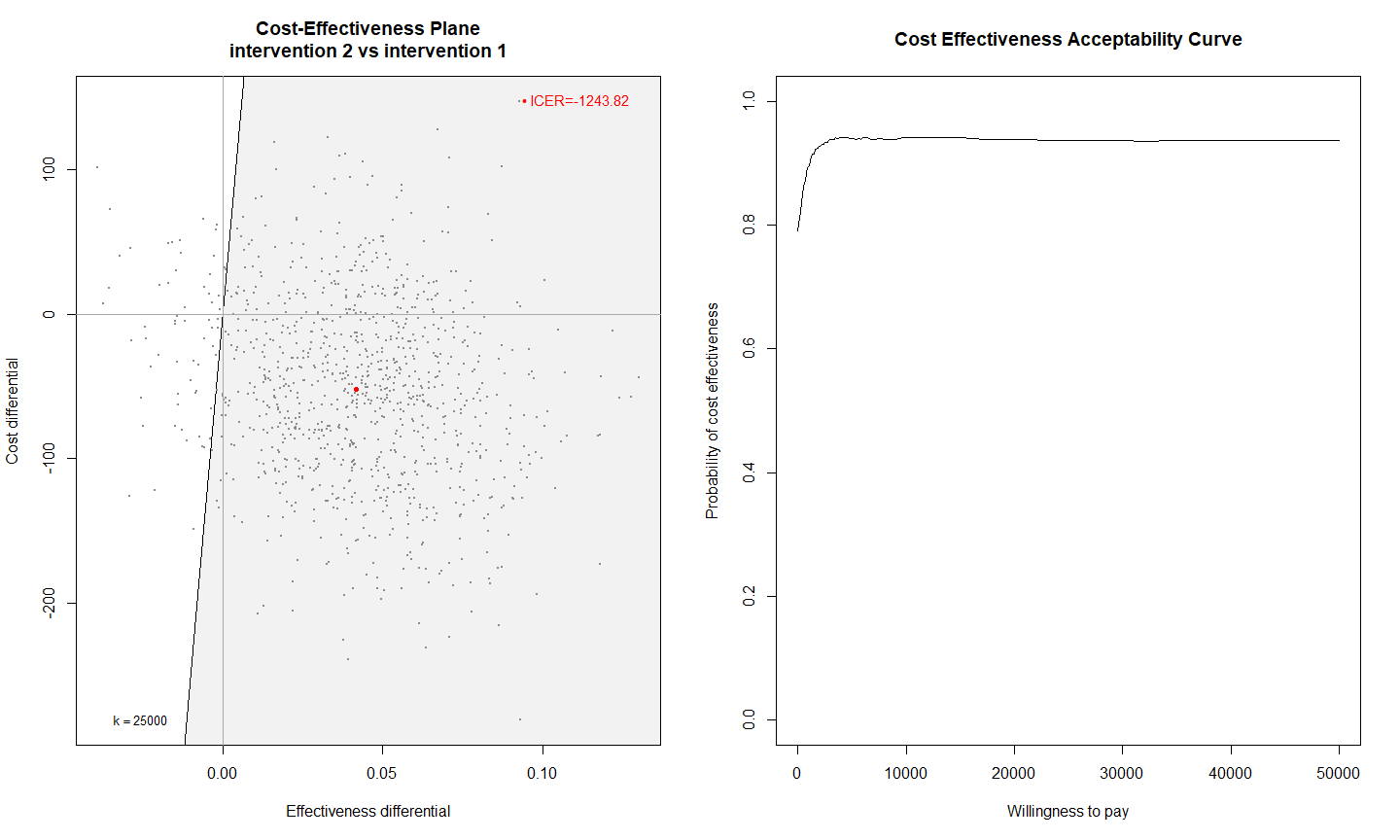For more information on how to interpret and customise these plots, see the BCEA package. ## Pattern mixture models The second type of missingness model available in missingHE are pattern mixture models, which can be fitted using the function pattern. These require the specification of two models. • The models are denoted with the terms model.eff and model.cost, and refer to the effectiveness and cost models in a similar way to what shown for selection in terms of related arguments, including the type of distributons and missingness assumptions. If the model is fitted under MAR, the argument Delta_e and Delta_c must be set to $$0$$. These are the priors on the sensitivity parameters which can be used to specify a MNAR assumption and that should therefore be removed under MAR. • However, in contrast to selection models, the models for $$e$$ and $$c$$ in pattern are fitted within each missingness pattern in the dataset. Patterns are defined only based on the number of individuals with observed and missing outcome data, for a total of $$4$$ maximum number of patterns. Parameters that cannot be identified from the data (because missing) are identified using some modelling restrictions. Two types of restrictions are available in missingHE: "CC" and "AC", which can be set using the argument restriction. The first identifies all unidentified parameters by setting them equal to the parameters estimated from the complete cases, while the second using those estimated from the available cases (patterns where the other outcome is missing). Type help(pattern) for more information on the assumptions of the model. • Although not directly accessible from pattern, the function implicitly fits a model for the probability of being associated with each missingness pattern in the data. This model is estimated using multinomial distributions and weakly-informative priors on all pattern probabilities. Posterior estimates for these parameters are then used to compute the weighted mean effects and costs across the patterns. > NN.pat=pattern(data = MenSS, model.eff = e ~ u.0, model.cost = c ~ e, + type = "MAR", restriction = "CC", n.iter = 1000, Delta_e = 0, Delta_c = 0, + dist_e = "norm", dist_c = "norm", ppc = TRUE) The model above assumes normal distributions for both outcomes under a MAR assumption and uses complete case restrictions to identify the parameters in each pattern. We can inspect the results by doing > coef(NN.pat, random = FALSE)$Comparator
$Comparator$Effects
mean    sd  lower upper
(Intercept) pattern1 0.177 0.141 -0.104 0.442
u.0 pattern1         0.790 0.152  0.500 1.101
(Intercept) pattern2 0.177 0.141 -0.104 0.442
u.0 pattern2         0.790 0.152  0.500 1.101

$Comparator$Costs
mean      sd     lower    upper
(Intercept) pattern1  240.664  50.559   141.894  341.652
e pattern1           -981.941 413.543 -1755.334 -146.819
(Intercept) pattern2  240.664  50.559   141.894  341.652
e pattern2           -981.941 413.543 -1755.334 -146.819

$Reference$Reference$Effects mean sd lower upper (Intercept) pattern1 0.666 0.075 0.515 0.809 u.0 pattern1 0.284 0.086 0.121 0.460 (Intercept) pattern2 0.666 0.075 0.515 0.809 u.0 pattern2 0.284 0.086 0.121 0.460$Reference$Costs mean sd lower upper (Intercept) pattern1 185.828 40.278 106.707 260.997 e pattern1 -179.191 351.793 -835.809 570.342 (Intercept) pattern2 185.828 40.278 106.707 260.997 e pattern2 -179.191 351.793 -835.809 570.342 which shows the presence of only two patterns in the dataset, given that estimates from only two patterns are displayed for each model. Note that estimates are exactly the same between the patterns, suggesting that one of the two is the complete case pattern and the other is formed by completely missing individuals (for whom estimates are set equal to those from the complete cases by setting restriction = "CC" inside pattern). Aggregted mean estimates over the patterns can be retrieved using print or, together with summary CEA results, using the summary command > summary(NN.pat) Cost-effectiveness analysis summary Comparator intervention: intervention 1 Reference intervention: intervention 2 Parameter estimates under MAR assumption Comparator intervention mean sd LB UB mean effects (t = 1) 0.873 0.017 0.846 0.901 mean costs (t = 1) 240.664 50.559 158.242 326.213 Reference intervention mean sd LB UB mean effects (t = 2) 0.917 0.023 0.88 0.953 mean costs (t = 2) 185.828 40.278 118.855 250.737 Incremental results mean sd LB UB delta effects 0.043 0.028 -0.005 0.092 delta costs -54.836 64.173 -155.426 53.818 ICER -1265.664  Standard graphical economic outputs based on the model results can again be obtained using functions from the BCEA package. ## Hurdle models The last type of missingness model that can be fitted in missingHE are hurdle models, implemented via the function hurdle. These require the specification of four models. • The first two are the models for $$e$$ and $$c$$ and are very similar to those used in selection. However, hurdle models are not technically speaking missingness models in that they do not allow to choose among specific missingness assumptions. They consist in two-part regressions designed to handle the presence of structural values in the data. The presence/absence of structural values in the effects and costs data can be specified in the function using the arguments se and sc. They must be set to NULL if the structural values in one of the outcomes is absent, and must be set equal to the actual structural value if these are present. • The last two models are fitted to the indicator variables associated with the presence or absence of a structural value for each individual in the data, denoted with the terms model.se and model.sc. These models estimate the probability of being associated with a structural effect and cost value using logistic regressions in a similar fashion to the models model.me and model.mc in selection. Once these probabilities are esitmated, the overall mean effects and costs in each arm are obtained through a weighted average between the means of the non-structural component (obtained from the models of $$e$$ and $$c$$) and the corresponding probabilities of having a structural value. • Due to the construction of hurdle models, the argument type takes different values compared to the standard MAR/MNAR assumptions in that the assumptions of the model are related to the probability of having a structural value rather than a missing value. missingHE allows to choose among Structural Completely At Random (SCAR) and Structural At Random (SAR) assumptions, the difference being the absence or presence of some covariate in the model for the structural probabilities. Within a Bayesian approach, hurdle models can be extended to impute missing values without the need of any ad-hoc imputation steps. We refer to help(hurdle) for more details on the assumptions behind hurdle models. > NN.hur=hurdle(data = MenSS, model.eff = e ~ u.0, model.cost = c ~ e, + model.se = se ~ 1, model.sc = sc ~ age, type = "SAR", se = 1, sc = 0, + n.iter = 1000, dist_e = "norm", dist_c = "norm", ppc = TRUE) The fitted model allows for the presence of structural ones in $$e$$ (se = 1) and zeros in $$c$$ (sc = 0) under a SAR assumoptions using age as a predictor for estimating the probability of having a structural value for both outcomes. We can extract the results from the regressions of $$e$$ and $$c$$ by typing > coef(NN.hur, random = FALSE)$Comparator
$Comparator$Effects
mean    sd  lower upper
(Intercept) 0.315 0.195 -0.077 0.708
u.0         0.617 0.220  0.165 1.052

$Comparator$Costs
mean      sd     lower   upper
(Intercept)  261.929  62.783   138.516 387.400
e           -759.380 486.114 -1656.729 184.005

$Reference$Reference$Effects mean sd lower upper (Intercept) 0.73 0.085 0.559 0.895 u.0 0.14 0.112 -0.081 0.354$Reference$Costs mean sd lower upper (Intercept) 257.846 43.939 168.379 341.323 e 38.304 400.528 -736.141 799.748 If interest is in the estimates for the parameters indexing the models of se and sc, the entire posterior distributions for these (as well as those of any other parameter of the model) can be extracted by accessing the elements stored inside the model_output list, available by typing NN.hur$model_output.

Finally, economic results can be summarised as

> summary(NN.hur)

Cost-effectiveness analysis summary

Comparator intervention: intervention 1
Reference intervention: intervention 2

Parameter estimates under SAR assumption

Comparator intervention
mean     sd      LB      UB
mean effects (t = 1)   0.907   0.02   0.874   0.939
mean costs (t = 1)   193.686 50.982 114.238 278.878

Reference intervention
mean     sd      LB      UB
mean effects (t = 2)   0.915  0.027   0.869   0.956
mean costs (t = 2)   186.536 40.441 121.785 252.568

Incremental results
mean     sd       LB     UB
delta effects    0.008  0.033   -0.044   0.06
delta costs      -7.15 65.692 -116.464 98.783
ICER          -868.657                       

and further exploration of the results can be done using the package BCEA.

## Model assessment

Before even looking at the results of the models fitted using selection, pattern or hurdle, it is recommended to check for possible issues in the convergence of the MCMC algorithm which, if present, may hinder the validity of the inferences. This is standard practice when fitting models based on iterative simulation methods, such as MCMC, where a larger number of iterations may be required to ensure the stability of the results.

missingHE allows to implement different types of convergence diagnostics for each type of model via the function diagnostic. For example, consider the selection model that we fitted before and saved into the object NN.sel. We can examine posterior density plots for the mean effects by treatment arm by typing

> diagnostic(NN.sel, type = "denplot", param = "mu.e", theme = NULL)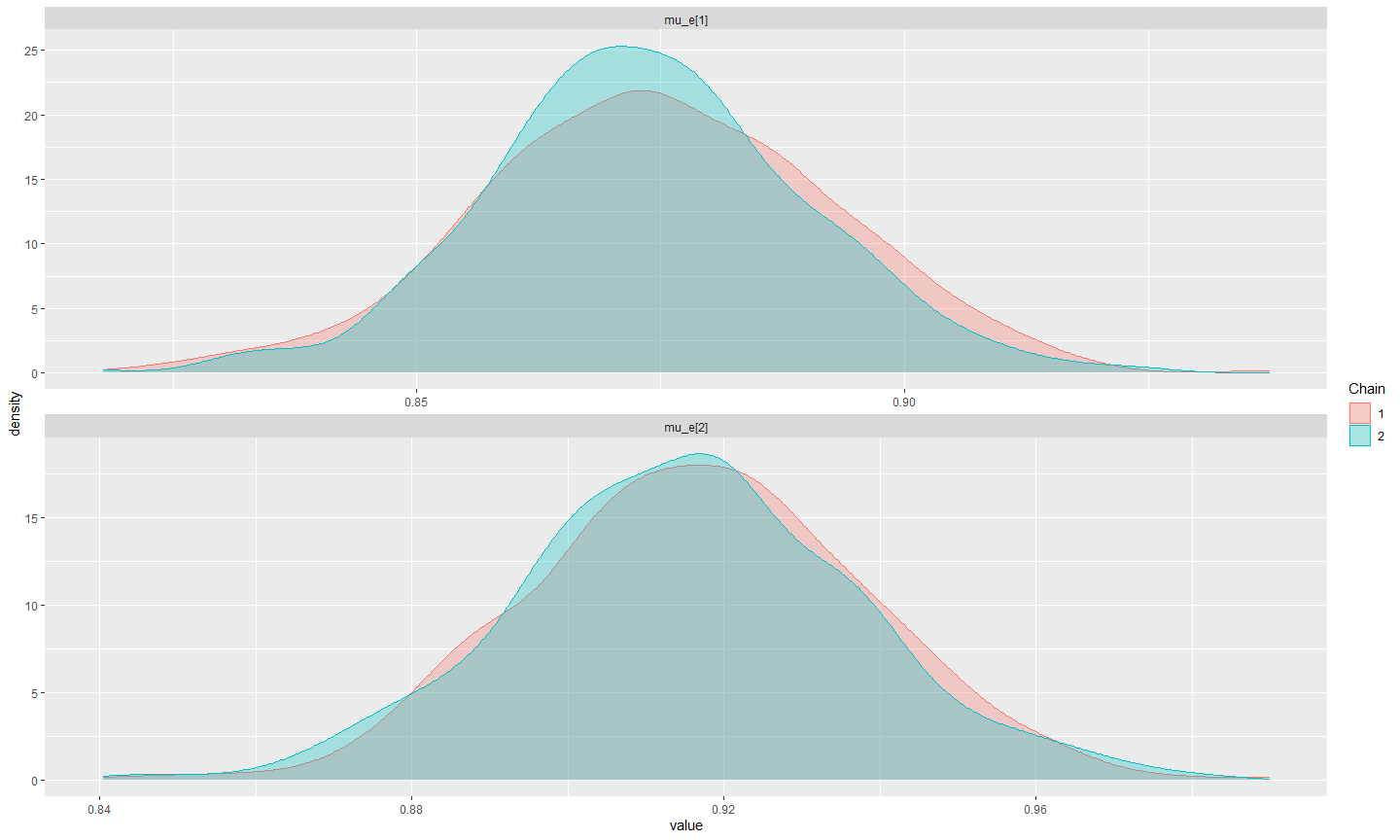The plots above do not indicate any potential issue in terms of failed convergence since estimates from both chains seem to overlap quite well (i.e. a single posterior distribution for each parameter seems to be reached).

1. The argument type is used to select the desired type of diagnostic measure (see help(diagnostic) for a list of all types available). For example, we can look at trace plots for the mean costs estimated from the pattern mixture model by typing
> diagnostic(NN.pat, type = "traceplot", param = "mu.c", theme = NULL)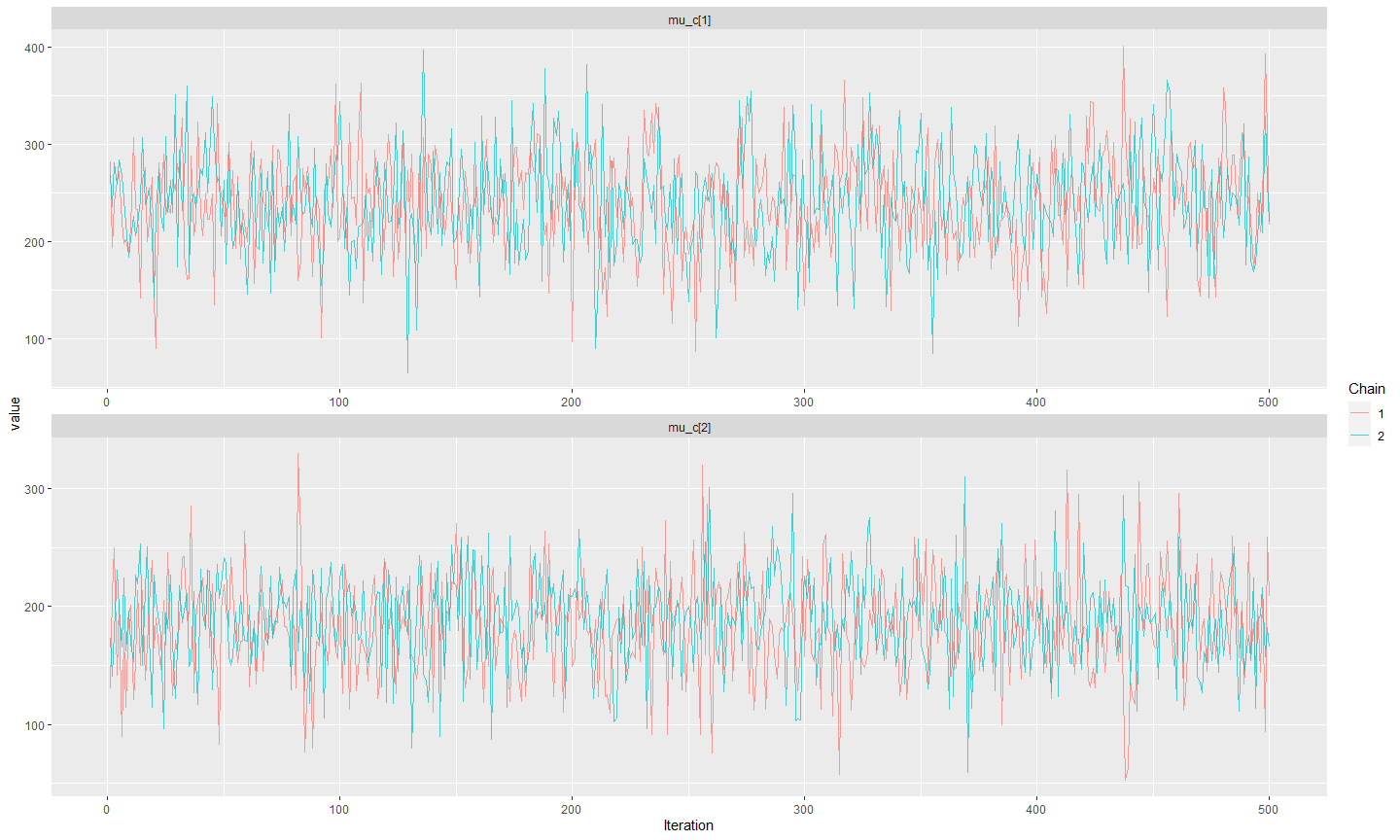1. The argument param denotes the parameter for which the diagnostics should be shown. The list of parameters that can be selected varies depending on the type of model fitted (e.g. selection or hurdle) and the assumptions made (e.g. MAR or MNAR). Type help(diagnostic) for the full list of parameters available for each type of model and assumptions. It is also possible to set param = "all" to display the diagnostic results of all parameters in the model together. For example, we can look at the autocorrelation plots for the posterior distribution of the probability of having a structural zero costs in the hurdle model by typing
> diagnostic(NN.hur, type = "acf", param = "p.c", theme = "base")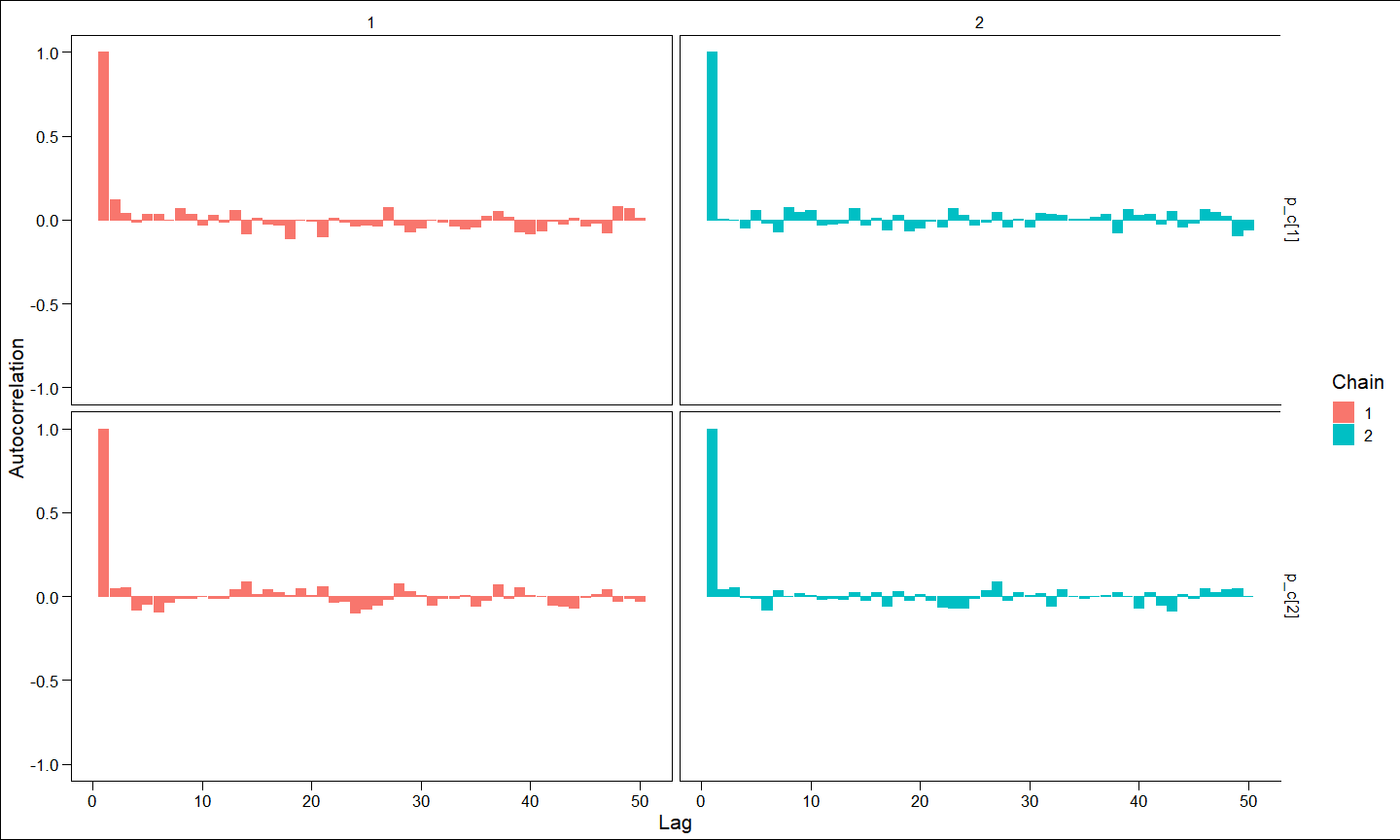1. The argument theme selects the type of backgroung theme to be used for plotting, chosen among a pre-defined set of themes whose names can be seen by typing help(diagnostic).

## Checking imputations

It is possible to look at how missing outcome values are imputed by each type of model using the generic function plot that, when applied to an object generated by missingHE functions, such as the model stored in NN.sel, produces the following output

> plot(NN.sel, class = "scatter", outcome = "all")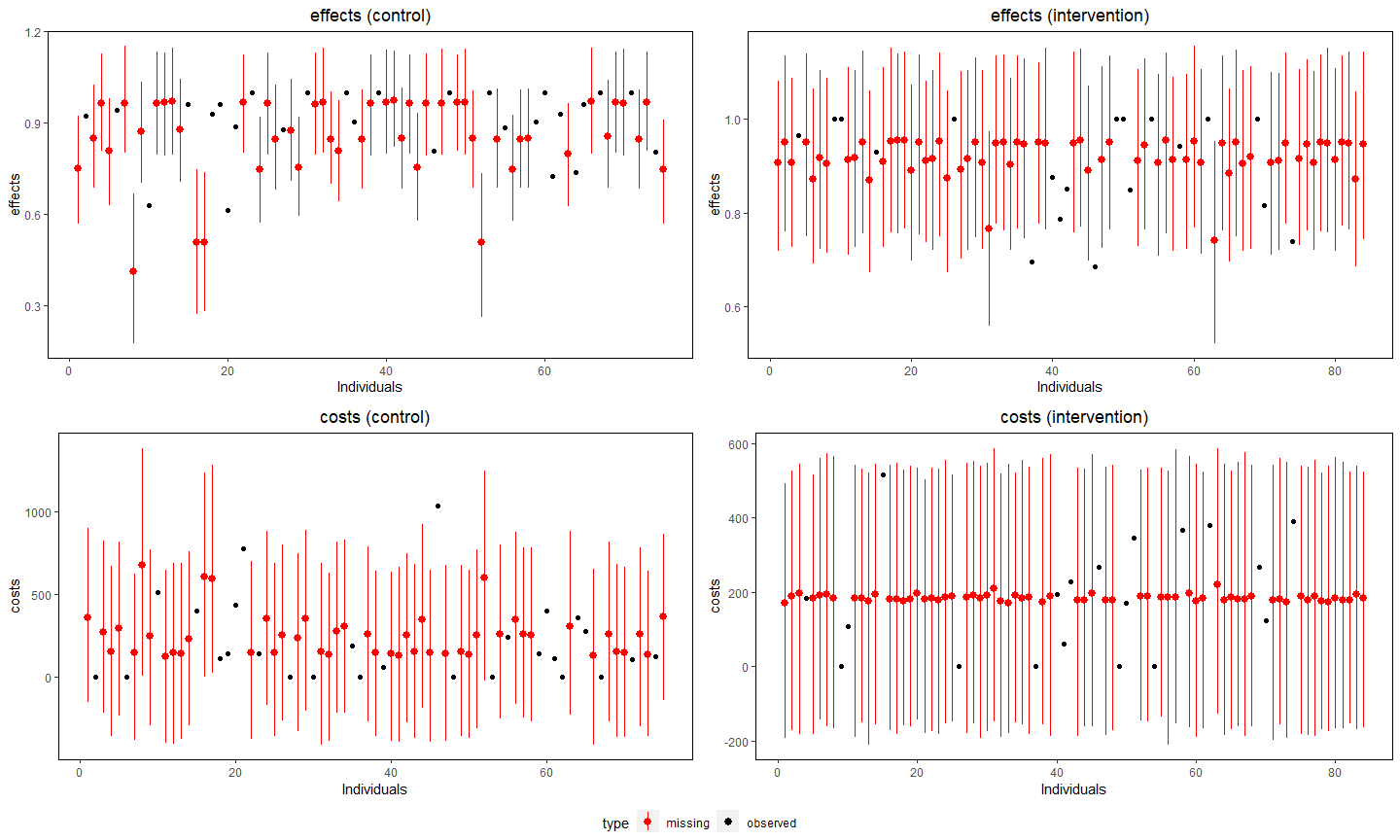The four plots show the observed values (black dots) and the posterior distribution of the imputed values (red dots and lines) by type of outcome (effects top, costs bottom) and treatment group (control left, intervention right).

1. The argument class specifies what type of graphical output should be displayed, either a scatter plot (scatter - default option) or a histogram (histogram). For example, we can show the histogram of the imputations produced by the pattern mixture model by typing
> plot(NN.pat, class = "histogram", outcome = "all")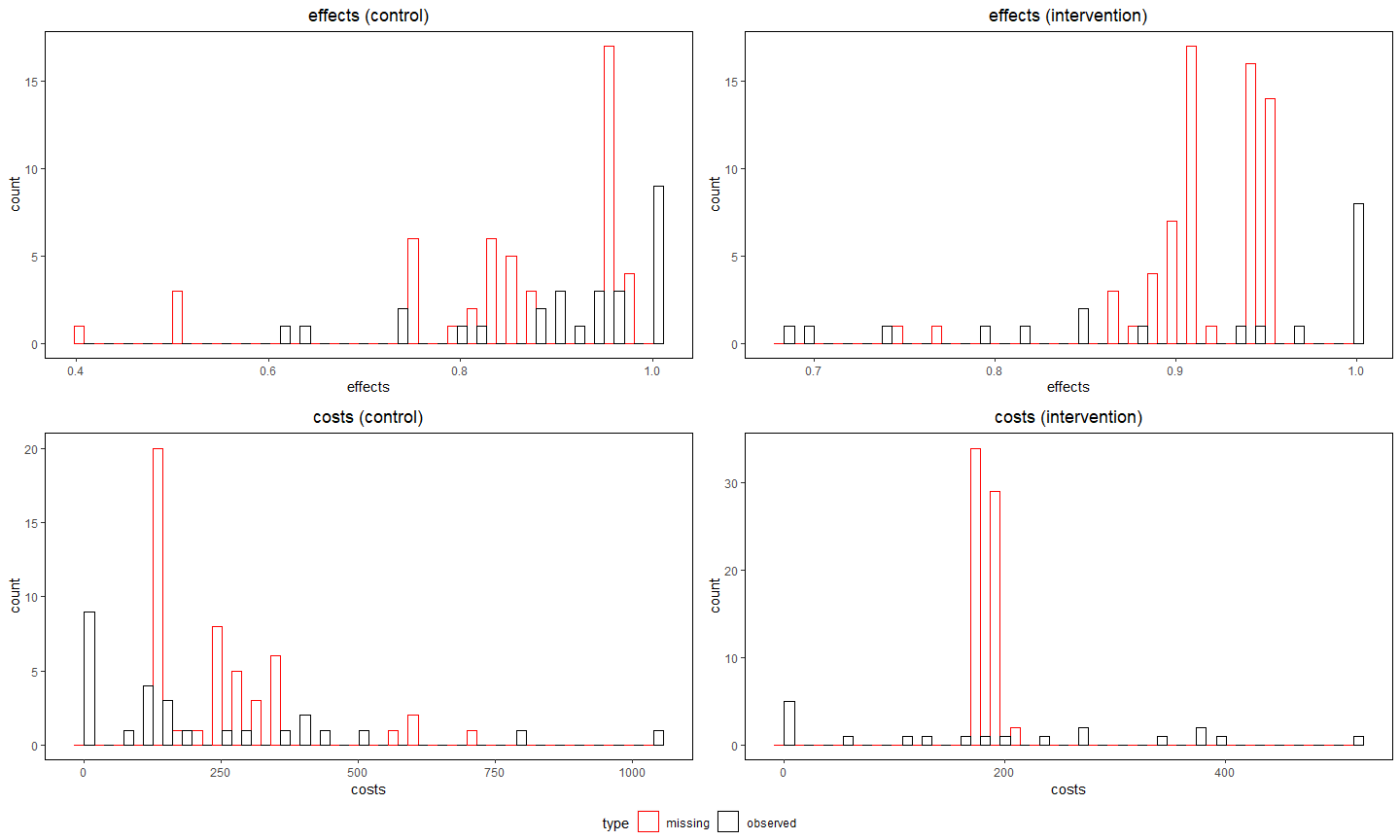1. The argument outcome specifies for which outcome the results should be shown. Available choices include either all variables in both groups (all - default), only the effects or costs variables (effects and costs), only the variables in a specific group (arm1 and arm2) or a combination of these. For example, we can look at the distributions of the imputed costs in the control group for the hurdle model by typing
> plot(NN.hur, class = "scatter", outcome = "costs_arm1")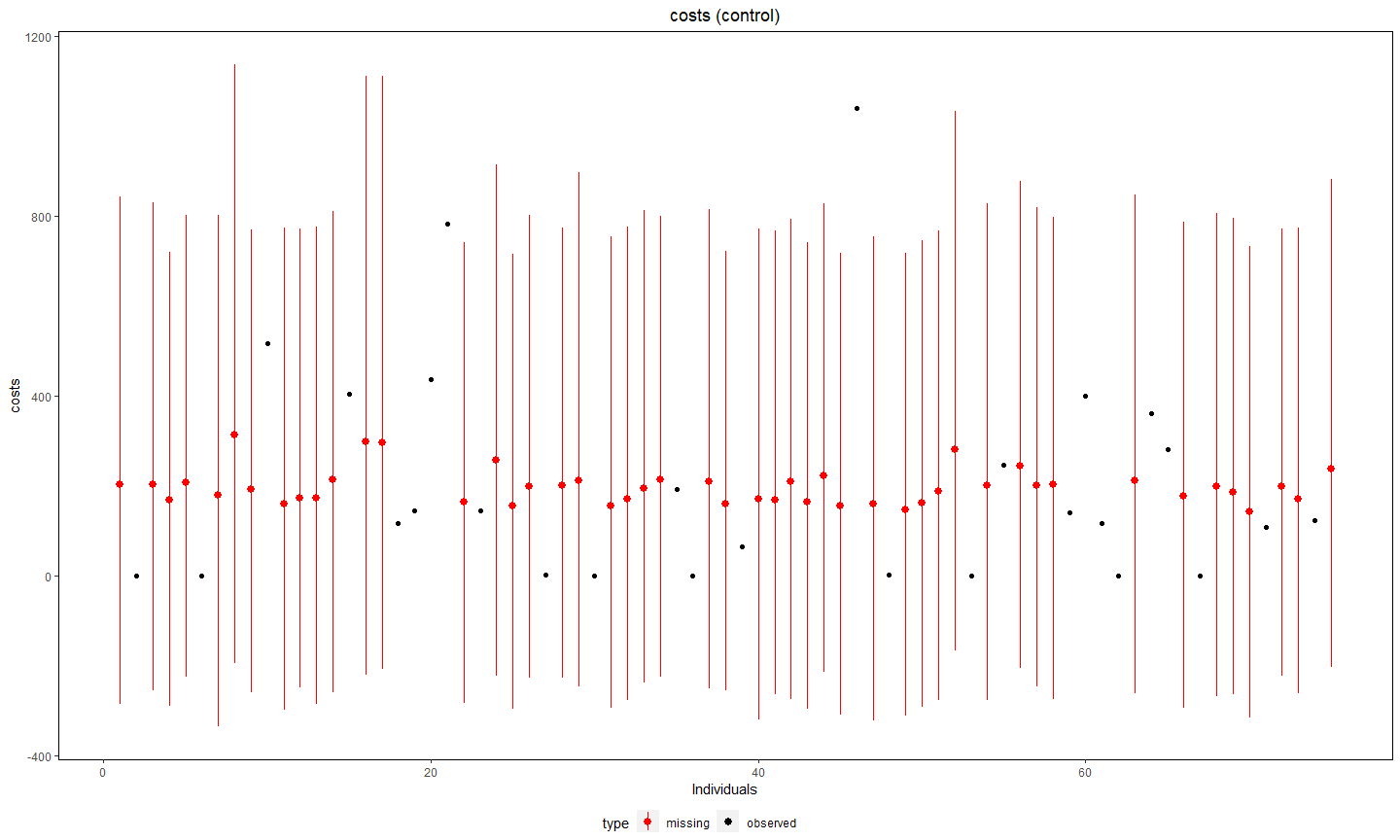## Model comparison and fit

We can check the fit of the models to the observed data by looking at posterior predictive checks (PPC) and compare the fit of altenative model specifications via preditive information criteria (PIC). Both measures are really useful when checking whether or not the results from the model align with the information from the observed data and for choosing the best models among those considered.

### PPC

The idea behind PPCs consists in using the estimated parameters from the model to generate replications of the data, which can then be compared with the observed values to detect possible inconsistencies in the replications. The main objective is to see whether the model is able to capture some aspects of the data which are of interest (e.g. mean, skeness, proportions of structural values, etc…), which would suggest a good fit of the model.

You can implement different types of checks in missingHE using the function ppc. For example, we can look at replicates of the histograms of the data for the effects in the control group based on the results of the selection model by typing

> ppc(NN.sel, type = "histogram", outcome = "effects_arm1", ndisplay = 8)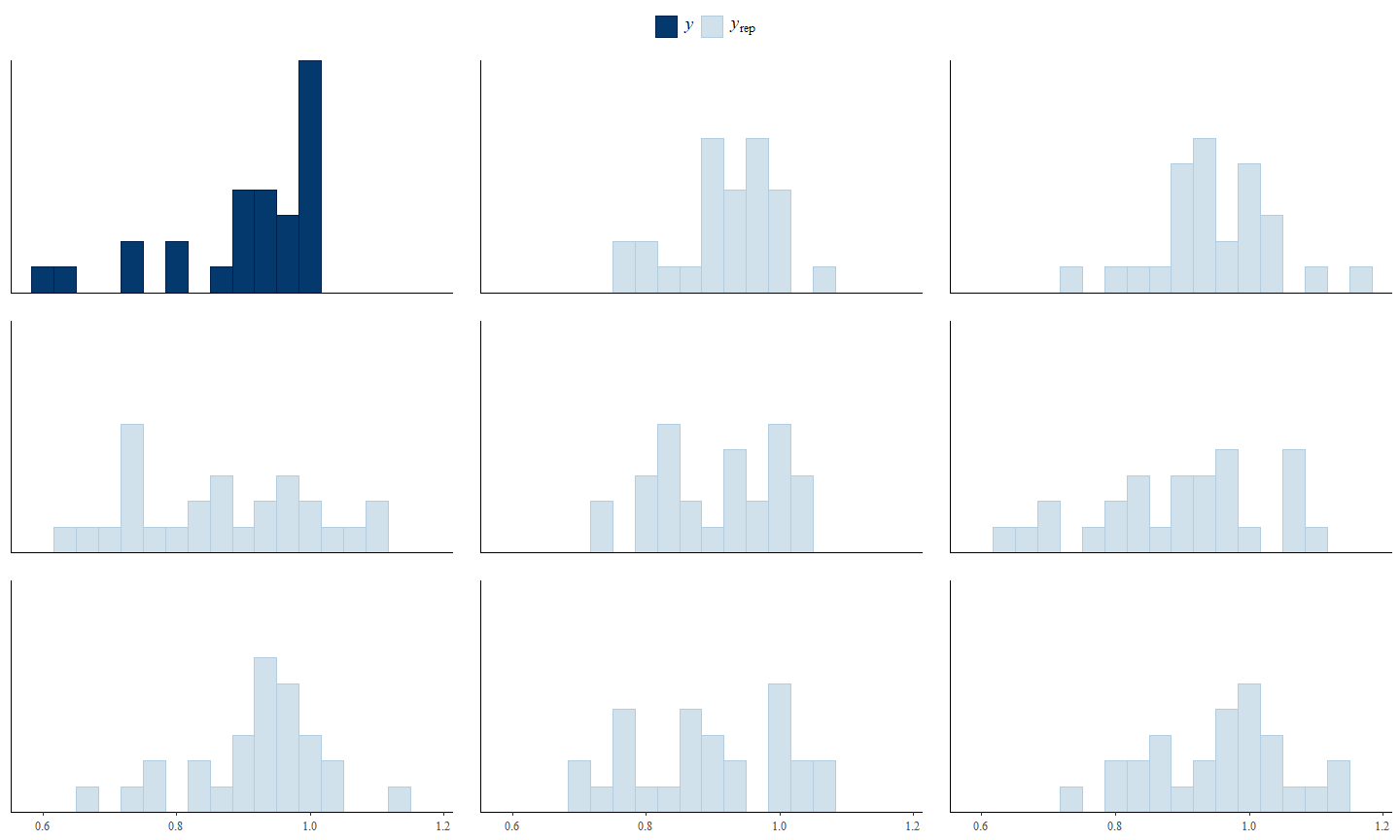1. The argument type selects the type of check to display. Different types of plots can be drawn using specific names. See help(ppc) for the full list of choices. The argument ndisplay indicates the number of replications that should be displayed for the comparison. For example, we can compare the observed and replicated kernel densities for the effects in the control group based on the results from the pattern mixture model by typing
> ppc(NN.pat, type = "dens", outcome = "effects_arm1", ndisplay = 8)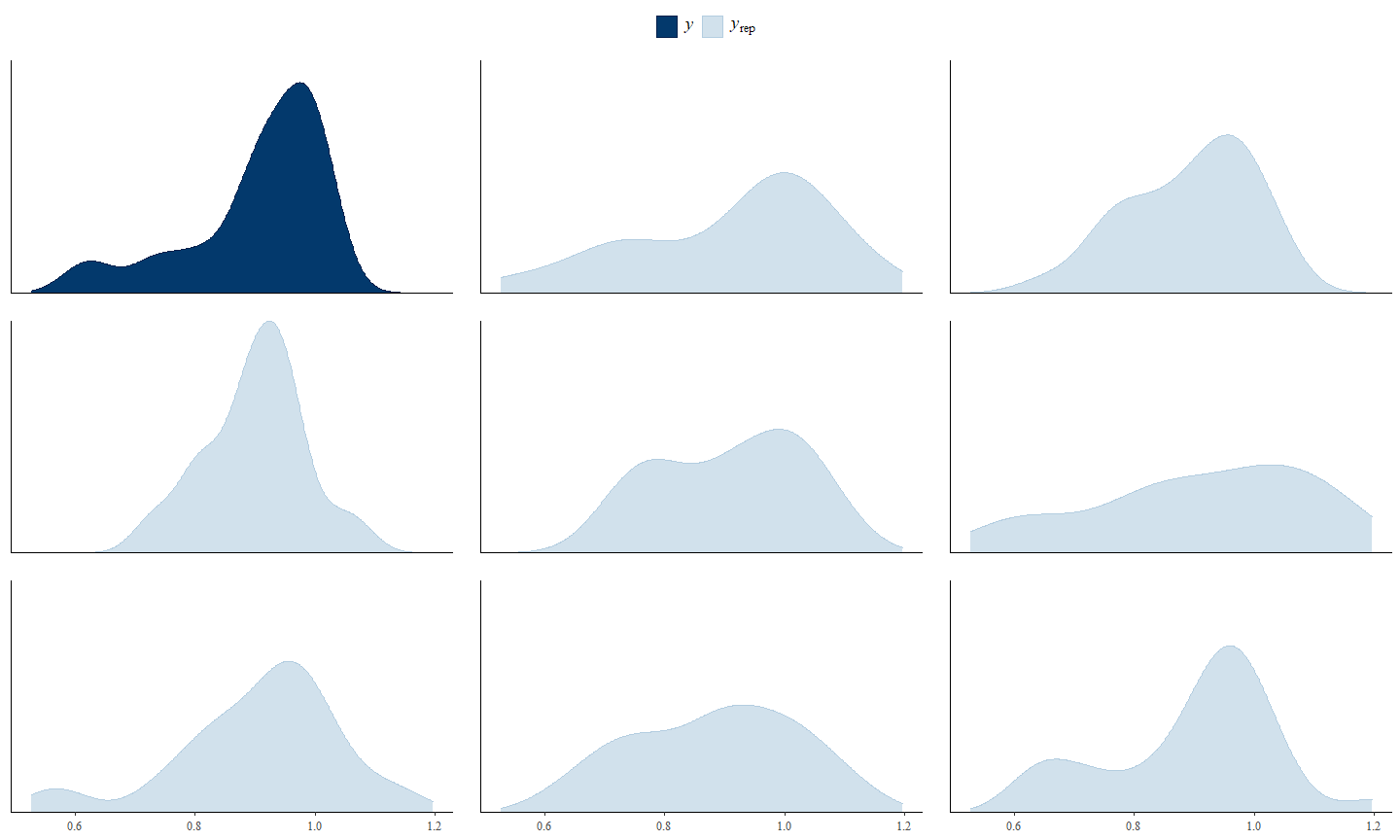1. The argument outcome chooses the type of variables for which results should be displayed. Available options include: all for both effects and costs in each treatment group, effects and costs for the corresponding outcomes in each group, and a combination of these. See help(ppc) for the list of all options. For example, we can look at overlayed densities between observed and replicated data for all variables based on the results from the hurdle model by typing
> ppc(NN.hur, type = "dens_overlay", outcome = "all", ndisplay = 25)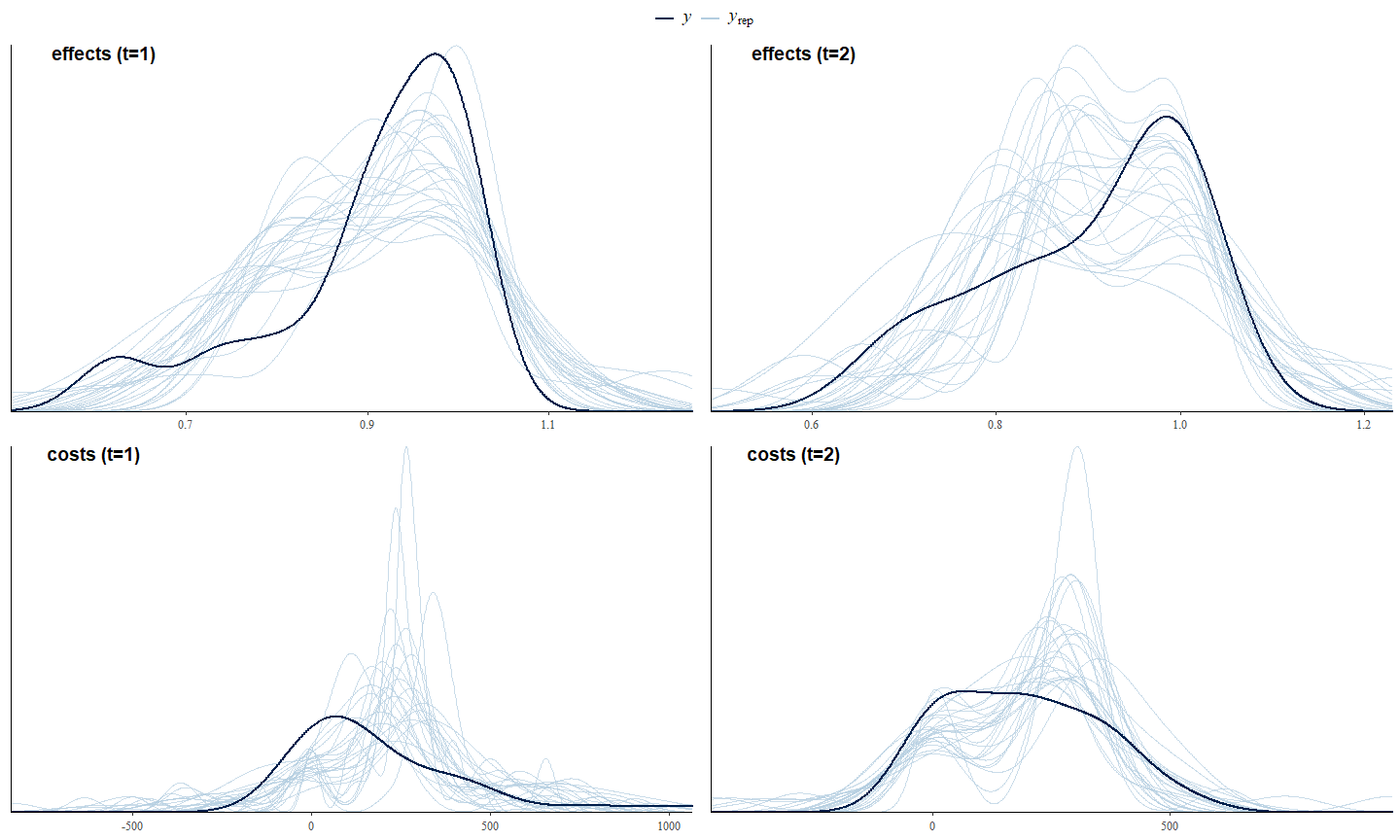### PIC

PICs compare the fit to the observed data form alternative model specifications in terms of a measure based on the loglikelihood of the model (deviance) and a penalty term for model complexity (effective number of parameters). The key message is that models associated with lower PIC values have a better fit to the observed data compared with models associated with higher PIC values. It is very important to remember that, when dealing with partially-observed data, the fit of the model can only be assessed based on the observed values. Thus, comparison by means of PICs is always partial since the fit to the unobserved values can never be checked. This is why it is generally not recommened to compare models fitted under MNAR assumptions as the comparison may be completely meaningless.

Three main types of PICs can be selected in missingHE via the pic function. Choices include: the Deviance Information Criterion (DIC), the Widely Applicable Inofrmation Criterion (WAIC), and the Leave-One-Out Information Criterion (LOOIC). Among these, the latter two are typically preferred as they are calculated on the full posterior distribution of the model and do not suffer from some potential drawbacks (e.g. reparameterisation of the model) that may instead affect the DIC. Type help(pic) for more details about these measures. For example, we can compare the fit to the observed data from the three models fitted using WAIC by typing

> pic_sel <- pic(NN.sel, criterion = "waic", module = "both")
> pic_pat <- pic(NN.pat, criterion = "waic", module = "both")
> pic_hur <- pic(NN.hur, criterion = "waic", module = "both")
>
> #print results
> c(pic_sel$waic, pic_pat$waic, pic_hur\$waic)
  539.2823  539.0758 -465.3983

The results indicate a much better fit of the hurdle model compared to the others, with a WAIC estimate which is negative. This is reasonable since hurdle models can capture the structural values which are instead ignored by selection or pattern mixture models. However, hurdle models do not allow the exploration of MNAR assumptions and therefore their results are entirely based on MAR.

The argument criterion specifies the type of PIC to use for the assessment, while module indicates for which parts of the model the measure should be evaluated. Choices are: total (default), which shoul be used for comparing models having the same structure; both, which uses both the models for $$e$$ and $$c$$ but not the auxiliary models (e.g. those for me and mc in selection); e or c, which use only the model for the effects or costs.